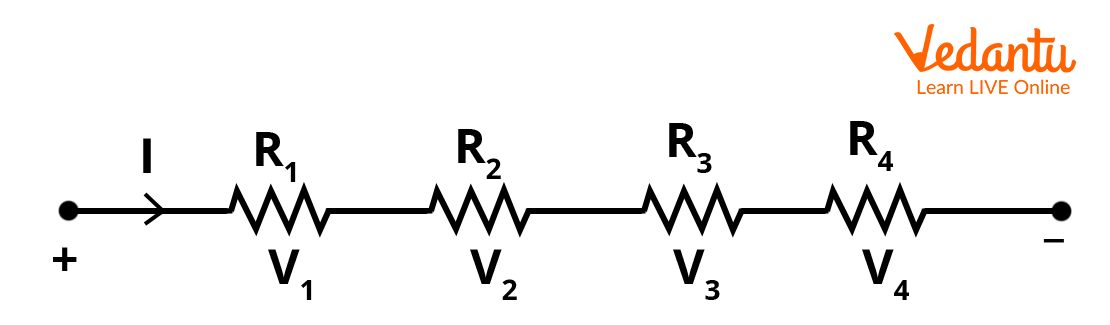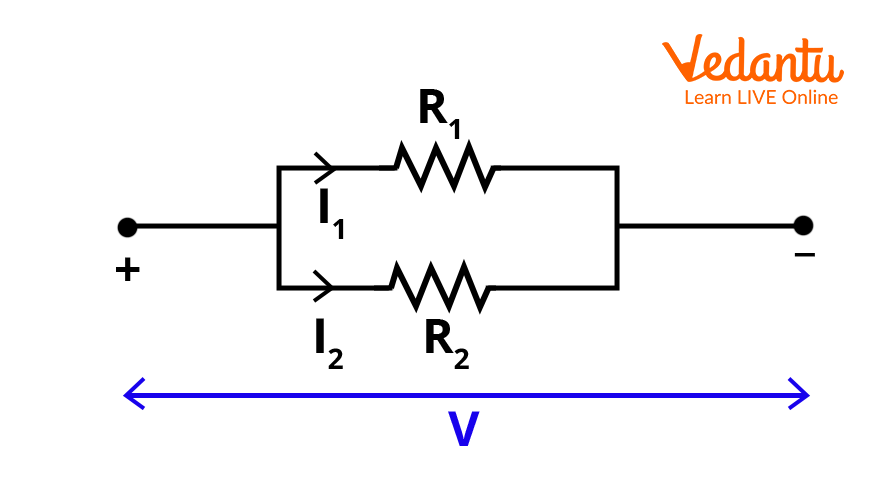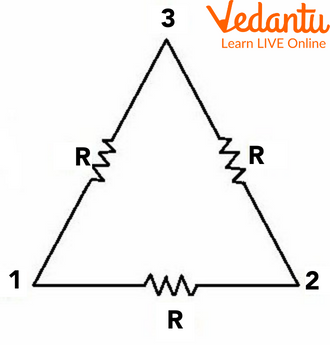Courses
Courses for Kids
Free study material
Free LIVE classes
More

# Equivalent Resistance for Series and Parallel Circuits - JEE Important TopicLIVE
Join Vedantu’s FREE Mastercalss

## Introduction to Equivalent Resistance

Before finding out what equivalent resistance is let's dive into the introduction of resistors. A resistor is a passive electrical component which resists the current flowing in a direction in an electric circuit. The measurement of how a resistor opposes the current flow in a circuit is known as electrical resistance. It is usually represented by the letter R. In physics, any material that we use in circuits possesses this electrical resistance. This resistance of a material is the reason why any conductor lets out heat energy when a current is passing through it.

As we already know there is a very important law that is to be discussed while learning the properties of resistance. That is Ohm's law. Therefore, ohm's law states that the current flowing through any conductor is directly proportional to the voltage potential across it.

I.e., $V\propto I$

Therefore, V = IR

The constant that resolves the proportionality here is called the resistance.

Where V is the potential difference across the conductor and I is the current flowing across it. The unit of resistance is ohms and it is represented by the symbol, Ω. Let us know what exactly the equivalent resistance is in detail in the following article.

## What is Equivalent Resistance?

Generally, when complex circuits are designed to control the current flow or the voltage difference in an electric circuit, the construction of the circuit would consist of numerous resistors in it. It looks intricate so there is a need to resolve it into shorter circuits and make it simple. This is why we calculate equivalent resistance to make the circuit simple with only one resistance representing the huge number of resistors. The equivalent resistance is between any two terminals or between any two node points of the circuit. When we replace all the resistors between two nodes or terminals, the determination of the equivalent current or the equivalent voltage becomes easier between those node points. And here, there are two types of resistor connections that are very essential to control the total resistance.

Those are series and parallel connections in an electric circuit. If we want to increase the total resistance of the circuit, then all the resistors are meant to be connected in series whereas, in order to reduce the value of equivalent resistance, the resistors in the circuit should be connected in parallel. Let us discuss how the equivalent resistance formula varies in series and parallel connections.

## Resistors in Series Connection

Let’s discuss how resistors are connected in series in an electrical circuit and how to find equivalent resistance for a series circuit.

Equivalent Resistance in Series Connection:

In a series circuit, all the resistors are placed consecutively and connected through wires. If a voltage source is connected at one terminal, then the current starts flowing in the circuit. In a series connection, the current flowing through every resistor is equal. There is only one path for the current to flow in the series circuit. Let's say there is a voltage source and 4 resistors are connected in series as shown in the below figure.Series Connection of Resistors

Here, the current starts flowing from the positive potential of the voltage source and passes through all the four resistors and then reaches the negative terminal of the voltage source. The current flows in an equivalent manner across all the resistors. The following is the equivalent resistance formula for the ‘n’ resistors connected in series:

Req = R1 + R2 + R3 + .....Rn

The above equation tells us that the equivalent resistance in a series circuit is the sum of all the resistances connected in series in an electrical circuit.

If there are more resistors connected in series, eventually the equivalent resistance increases as it is the sum of all the resistances in series. If the total resistance of the circuit is high, then it offers high resistance to the flow of the current. This in turn reduces the total current flowing in the circuit. The above explanation can be interpreted with the ohm's law.

Where V = IR

And $I=\dfrac{V}{R}$

Therefore, the current flowing in a circuit is inversely proportional to the total resistance of the circuit.

## Resistors in Parallel Connection

In a parallel circuit, the resistors are connected in a parallel manner in branches of a circuit. Two resistors of a circuit are said to be parallel if they are in between the same ends as shown in the figure.Two resistors in parallel

## Equivalent Resistance in Parallel Connection:

In a parallel circuit,  the current gets divided into the branches which are parallel but the potential difference is the same across all the resistors which are in parallel because they are present in between the same nodes. The below-given equation is the total equivalent resistance for the n resistors connected in parallel.

$\dfrac{1}{R_{eq}}=\dfrac{1}{R_{1}}+\dfrac{1}{R_{2}}+\dfrac{1}{R_{3}}+----\dfrac{1}{R_{n}}$

As you can see, the total resistance is made smaller by connecting the resistors in parallel.

## Triangle Resistors Equivalent Resistance:

Let us understand with the help of triangle resistors equivalent resistance concept.  Let’s say three resistors are connected in the form of a triangle where the resistance of the three resistors is equal to R as shown in the below image.Triangle Resistors Equivalent Resistance

Here, let’s consider the base nodes 1 and 2. In between nodes 1 and 2, there are two paths for resistors, 12 and 132. So these two would be parallel.  Therefore the two resistors in path 132 are connected in series, the equivalent resistance is R + R = 2R.

Now, this 2R resistor is in parallel with the third resistor in between nodes 1 and 3.

$\dfrac{1}{R_{e q}}=\dfrac{1}{R_{1}}+\dfrac{1}{R_{2}}$

Where R1 = R and R2 = 2R

Therefore,

### \begin{align} & \dfrac{1}{R_{\mathrm{eq}}}=\dfrac{1}{R}+\dfrac{1}{2 R}=\dfrac{3}{2 R} \\ &R_{\mathrm{eq}}=\dfrac{2 R}{3} \end{align}

This is the equivalent resistance formula when the three resistors of equal resistance R are connected in the shape of a triangle.

There are some methods used like nodal analysis or a mesh analysis when there are many complex circuits where it is difficult to find whether the resistors are in series or parallel. For example, finding the equivalent resistance of a cube and even in Wheatstone bridge equivalent resistance. The equivalent resistance of a cube is found through an analysis method rather than using these simple methods.

Concepts discussed can be better understood by the help given numerical example

Example - If two resistors with resistance, R1 = 2 $\Omega$ and R2 = 4 $\Omega$ are connected in parallel to which a resistor of resistance R3 = 3 $\Omega$ is connected in series. Find the equivalent resistance?

Ans: As the resistors, R1 and R2 are connected in parallel, the equivalent resistance for these two resistors is given by the equation,

\begin{align} &\dfrac{1}{R_{p}}=\dfrac{1}{R_{1}}+\dfrac{1}{R_{2}}=\dfrac{1}{2}+\dfrac{1}{4}=\dfrac{3}{4} \\ &R_{p}=\dfrac{4}{3} \end{align}

Now, this parallel equivalent resistance is in series with a $3\Omega$ resistor, Therefore the total equivalent resistance is,

$R_{e q}=\dfrac{4}{3}+3=\dfrac{13}{3} \Omega$

This is the equivalent resistance of the circuit. Let us conclude what we have learnt till now.

## Conclusion

In short, the equivalent resistance could be described as the total electrical resistance that the total number of resistors offer against the voltage source present in the circuit. Therefore, we discussed how to find equivalent resistance when the resistors are in series or parallel. These formulae are very useful in finding the total resistance of a circuit. We learnt how resistance is being controlled by placing them in series connection or parallel connection circuits through this article.

Last updated date: 20th Sep 2023
Total views: 132k
Views today: 3.32k

## FAQs on Equivalent Resistance for Series and Parallel Circuits - JEE Important Topic

1. What is the weightage of this topic in JEE?

This topic is very important and it is often addressed as the sub-concept of current electricity. The types of questions asked are to find the equivalent resistance of the circuit which consists of a mixed connection of series and parallel and to find the current flowing through a specific resistor or the potential difference across the resistor. A maximum of three questions could be asked from the current electricity and the weightage is 10% for this topic in JEE.

2. What are the factors that affect the electrical resistance of a conductor?

There are certain factors on which the electrical resistance of a conductor depends. If any of the factors changes due to any cause, then the electrical resistance changes too. The factors are the length of the conductor, the cross-sectional area of the conductor, the material with which the conductor is made up and the temperature of the conductor.

There is a relation between the length, area and the resistance of a conductor which is $R=\rho \dfrac{L}{A}$

Where the resistance is directly proportional to the length of the conductor and inversely proportional to the cross-sectional area of the conductor.